Zoom SettingsThe size of the coordinate plane is defined by Xmin, Xmax, Ymin, and Ymax, as seen at the left.

Xmin must be less than Xmax
Ymin must be less than Ymax
Xscl and Yscl define the
distance between tick marks
on the x-axis and y-axis
• To turn off tick marks,
let Xscl = 0 and Yscl = 0
•  3/2 aspect ratio in windowXres sets pixel resolution 1 to 8.  The default is 1.  At Xres = 1, functions are evaluated and graphed at each pixel on the x-axis.  At Xres = 8, at every eighth pixel along the x-axis.

Zoom - Zoom - Zoom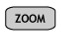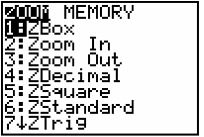#1 ZBox - draws a box to define the new smaller viewing window.  Move cursor to upper left corner of where your box will be placed.  Hit  ENTER to anchor that corner.  Move cursor to lower right corner of the desired box, press ENTER.  The box will fill the screen.#2 Zoom In - magnifies the part of the graph surrounding the cursor location.  Move cursor to the location you wish to magnify and hit ENTER.  To magnify a second time, hit ENTER again.  You can move the cursor between zooms and continue magnifying.  To return to previous graph, chooose #3 Zoom Out and press ENTER.  To quickly return to the 10 x 10 screen, press #6 Zstandard. #4 ZDecimal - plots the function with values Xmin = -4.7, Xmax = 4.7, Ymin = - 3.1, Ymax = 3.1.  The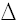X andY are 0.1.  In plain English, you have a smaller screen which traces nicely in increments of one decimal place. #5 ZSquare -X =Y.  The aspect ratio is adjusted so that circles will look like circles.  The domain in the window is -15 to 15 and the range is -10 to 10. #6 Zstandard - 10 x 10 window.  This window is usually large enough to show a representative portion of a graph.  Remember, however, that the graph is slightly distorted because the x and y units are not equal. #7 Ztrig - window appropriate for trig graphs.  In Radian Mode, the domain is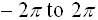, the range is -4 to 4, and Xscl =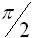.  In Degree Mode, the domain is -352.5° to 352.5°, the range is -4 to 4, and Xscl = 90°. #8 ZInteger  -X =Y = 1.  Xscl, Yscl = 10.  Each pixel represents one unit along the horizontal and vertical axes.  Move cursor to desired center, ENTER. #9 ZStat - redefines window to appropriate size for statistical plotting.  Automatically adjusts the window to show all data points in the active plot in STATPLOT. #10 ZFit - recalculates Ymin and Ymax using current x-values to show best graph.  The range is adjusted so that the function will enter the window at a corner on the left side.  If the function is odd, it will leave the window at the other corner of the diagonal.  If the function is even, the range will cover the extreme y-value in the other direction of the entry point.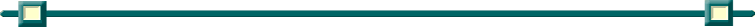Finding Your Way Around TABLE of  CONTENTS